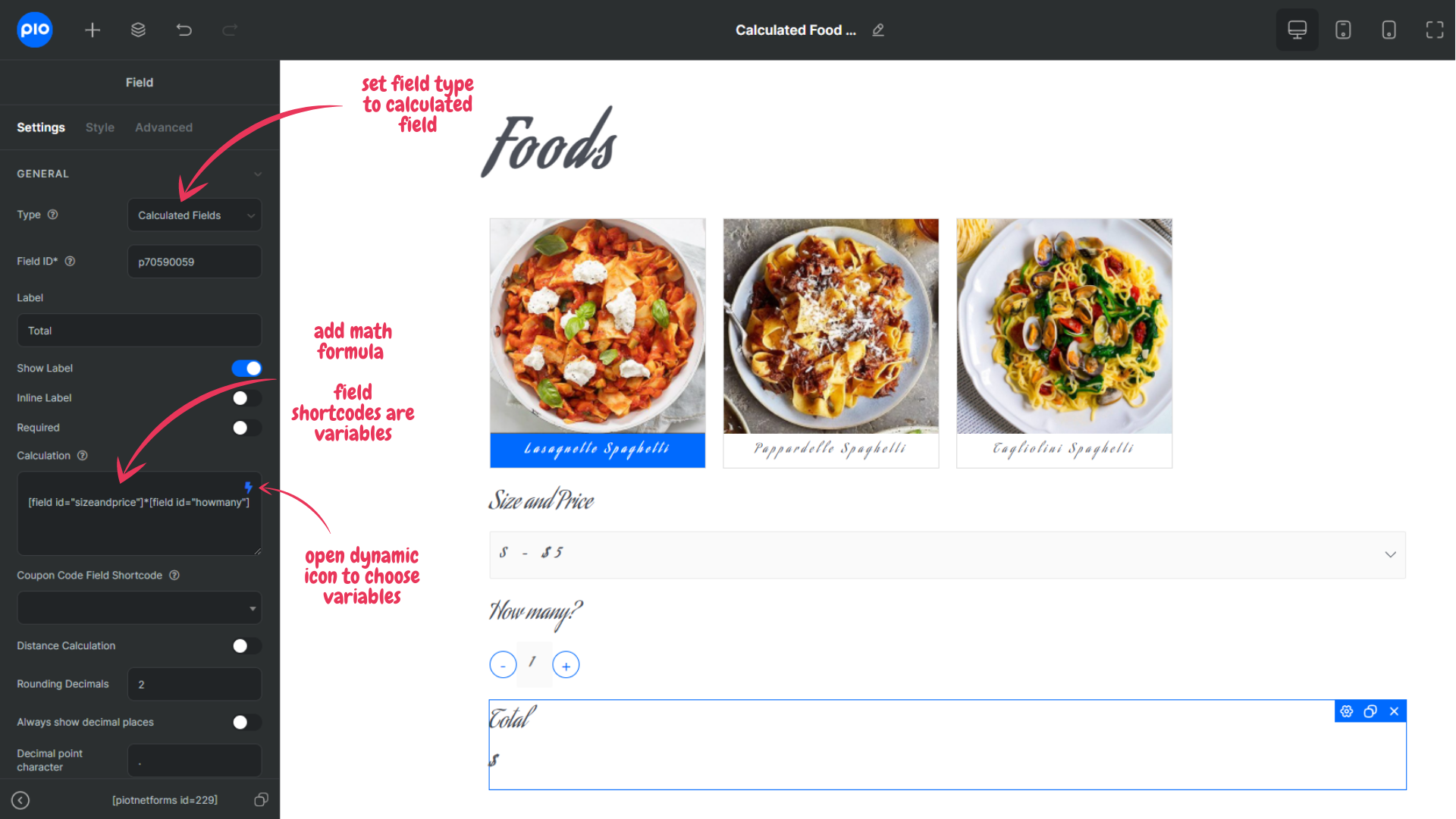HIDE
Basic Features

# Calculated Field

In this article, you will learn how to use a calculated field to create calculations in forms.
The feature supports various mathematic operators for many calculating needs.

## Food Order Calculation Example

This is an example of applying a calculated field in the Bill Calculation form.
The actions are:
“Customers select Spaghetti type, size, quantity then the form calculates total price”

Demo

\$

To do this:

• Step 1: Create a form with all fields: Foods – select field, Size, and price – select field, How many? – number field and Total – calculated field.
• Step 2: Edit the Total – calculated field, and set the math formula:
[Size and Price field]*[How many? Field]
Shortcode of the fields will be used as variables.Now the form is ready. After customers select product, Size and Quantity, the Calculated field will automatically calculate the total amount.

## Available Math Functions

A calculated field is a powerful feature of Piotnet which helps you to do mathematic formulas in forms.
The feature is widely applied in quotation generation.
Refer to this document to know the available operators and object that the field can perform.